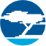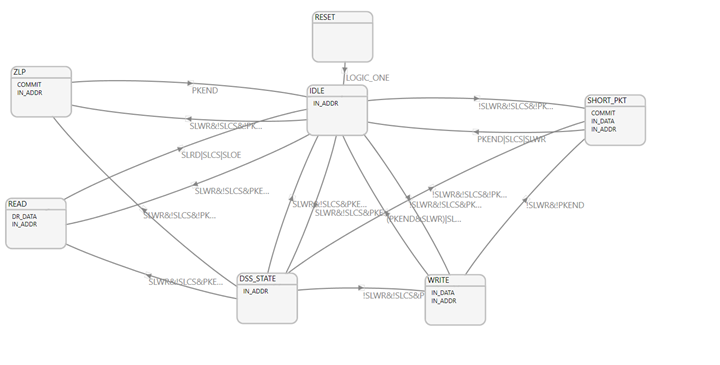# CYU3P_GPIF_ERR_INVALID_STATE GPIF Error in FX3 SDK – KBA232781Employee
EmployeeVersion: **

Question:
Why does the CYU3P_GPIF_ERR_INVALID_STATE GPIF error occur in FX3 SDK?

The GPIF error CYU3P_GPIF_ERR_INVALID_STATE occurs when the state machine reaches an invalid state. An invalid state is a state that is not defined the .h file generated by the GPIF II designer tool. Mostly, this happens when mirror states are used in the state machine. To know more about mirror states, see section 4.5 – GPIF II constraints of the GPIF Designer User Guide (gpif2_designer_userguide.pdf). This document is available with the FX3 SDK and can be found in the following location after installing the SDK: C:\Program Files (x86)\Cypress\EZ-USB FX3 SDK\1.3\doc\GPIFII_Designer.

When mirror states are used in the state machine, the state numbers of all mirror states associated with a state will not be defined in the .h file generated by the GPIF II designer tool. So, in a state machine that makes use of mirror states, if at any point in time the state machine reaches any mirror state, there are chances for the GPIF error CYU3P_GPIF_ERR_INVALID_STATE to be triggered. This will not affect the functionality of the state machine.

Another possible reason for the GPIF error CYU3P_GPIF_ERR_INVALID_STATE is also associated with the use of mirror states. Figure 1 illustrates the  reason with the  example of Slave FIFO state machine provided with AN65974.

Figure 1. Slave FIFO State MachineThe slave FIFO state machine in Figure 1 uses SLWR and PKEND for identifying mirror states. While building the state machine, the GPIF II designer warns that SLWR and PKEND are removed from the outgoing transition equations from the state IDLE and the state  DSS_STATE. If SLWR and PKEND are removed from the outgoing transition equations from the IDLE state, then the following are obtained:

1. IDLE -> READ : Equation: !SLCS & !SLRD & !SLOE when (WR,PKEND) = (1,1)
2. IDLE -> WRITE : Equation: !SLCS & SLRD when (WR,PKEND) = (0,1)
3. IDLE -> SHORT : Equation: !SLCS & SLRD when (WR,PKEND) = (0,0)
4. IDLE -> ZLP : Equation: !SLCS &SLRD when (WR,PKEND) = (1,0)

This means that the transition equation from the IDLE state and its mirror states can be either (!SLCS & !SLRD & !SLOE) or (!SLCS and SLRD).

Now, assume that the DSS_STATE is not used in the state machine. If you initiate a read, by first asserting SLCS and then asserting SLRD in the next clock cycle, it might cause a problem. The transition equation (!SLCS & SLRD) will be matched in the first clock cycle and the state machine will transition to an INVALID state. This is because for a read operation, (WR,PKEND) will be (1,1). When (WR,PKEND) = (1,1), the state machine can jump to a valid state only if the transition equation is !SLCS & !SLRD & !SLOE. Since this is not satisfied, the state machine will jump to an INVALID state.

The only way to avoid this problem is to place a valid state in the location matching the "!SLCS & SLRD" equation when (WR,PKEND) = (1,1). This is the DSS_STATE state in the state machine. If this DSS_STATE state is not present in the state machine, it will trigger the GPIF error CYU3P_GPIF_ERR_INVALID_STATE.

So, while developing a custom state machine that makes use of mirror states make sure that the state machine can transition to a valid state for any combination of inputs to avoid CYU3P_GPIF_ERR_INVALID_STATE. If required, a valid state (like DSS_State in AN65974) may be used.

605 Views